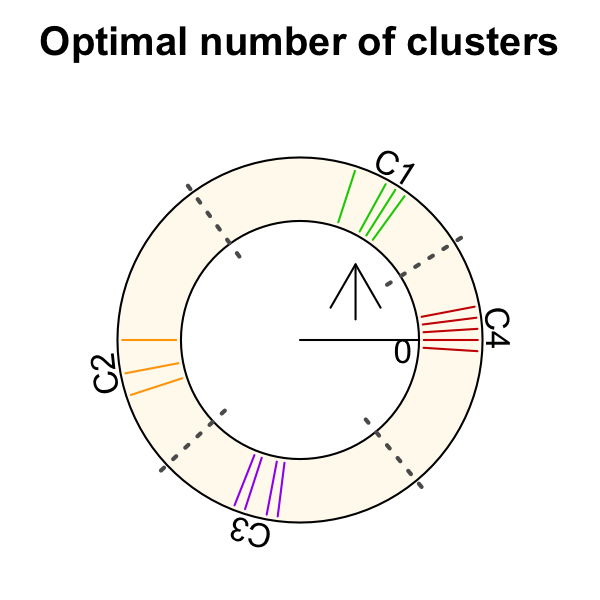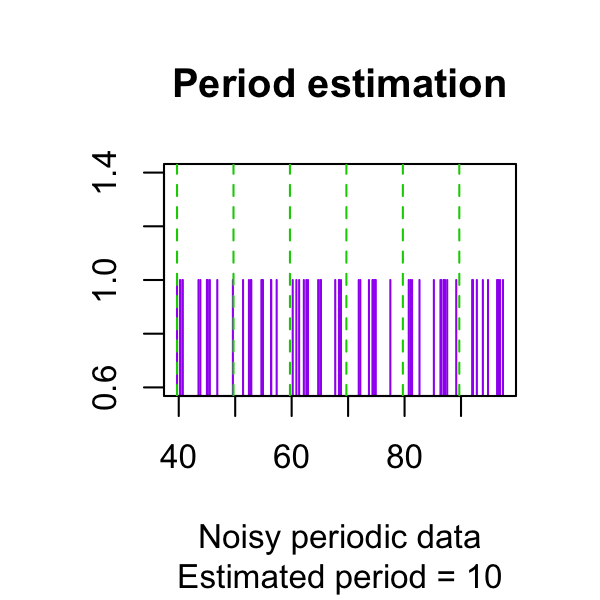# Circular Silhouette Examples

#### March 30, 2022

In this tutorial, we demonstrate how to calculate circular silhouette and apply it to find the optimal number of circular clusters and estimate the period of noisy periodic data.

## 1. Circular silhouette on clustered data

Given a set of circular data points and their cluster lables, we can compute the circular silhouette as follows:

library(CircularSilhouette)
o=c(19,0,4,6,10,12,15)
c=c(1,1,2,2,2,2,2)
circumference=20
silhouette=circular.sil(o, c, circumference, method="linear")
# print(silhouette)
knitr::kable(silhouette, col.names="Circular silhouette")
Circular silhouette
0.3165926

## 2. Finding an optimal number of clusters

On circular data, we can use maximum silhouette to select an optimal number of clusters. This requires a circular clustering algorithm. Here we use the R package OptCirClust. We will examine every value of $$k$$ in a given range of number of clusters. We select $$k$$ that maximizes the corresponding silhouette information.

library(OptCirClust)

Circumference=100
O=c(99,0,1,2,3,15,16,17,20,50,55,53,70,72,73,69)
K_range=c(2:8)
k <- find.num.of.clusters(O, Circumference, K_range)
result_FOCC <- CirClust(O, k, Circumference, method = "FOCC")
opar <- par(mar=c(0,0,2,0))
plot(result_FOCC, main="Optimal number of clusters",
sub=paste("Optimal k =", k))
par(opar)## 3. Estimating the period of noisy periodic data

Here we show that we can use circular silhouette and clustering to estimate the period of noisy periodic data. We have developed a preliminary function estimate.period() to estimate the period of noisy periodical signal. The possible periods provided by the function should be close to the true period. This is not ideal and we are improving the design to be more robust.

x=c(40,41,41,42,44,45,45,46,46,46,47,50,51,51,52,54,55,55,56,56,56,57,
60,61,61,62,64,65,65,66,66,66,67,70,71,71,72,74,75,75,76,76,76,77,
80,81,81,82,84,85,85,86,86,86,87,90,91,91,92,94,95,95,96,96,96,97)
set.seed(111)
x <- x + rnorm(length(x))

periodrange=c(80:120)/10
period<-estimate.period(x, periodrange)

cat("The estimated period is", period, "\n")
#> The estimated period is 10

plot(x, rep(1, length(x)), type="h", col="purple",
ylab="", xlab="Noisy periodic data",
main="Period estimation",
sub=paste("Estimated period =", period))
k <- (max(x) - min(x)) %/% period
abline(v=min(x)+ period * (0:k), lty="dashed", col="green3")